Successfully reported this slideshow.
We use your LinkedIn profile and activity data to personalize ads and to show you more relevant ads. You can change your ad preferences anytime.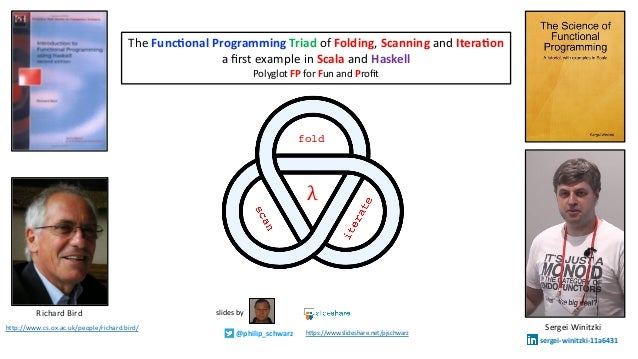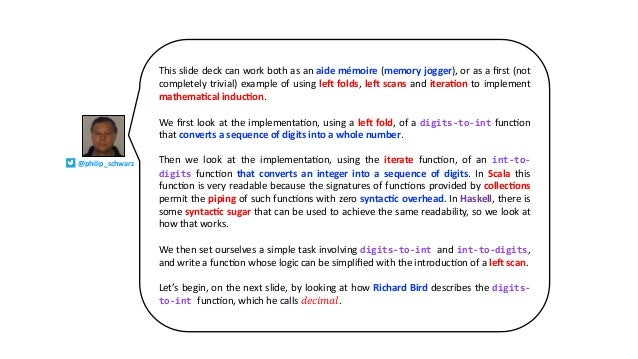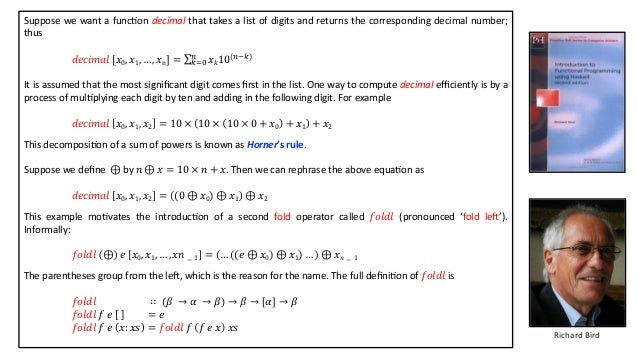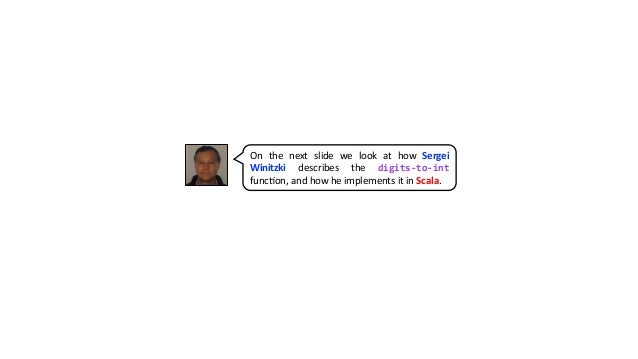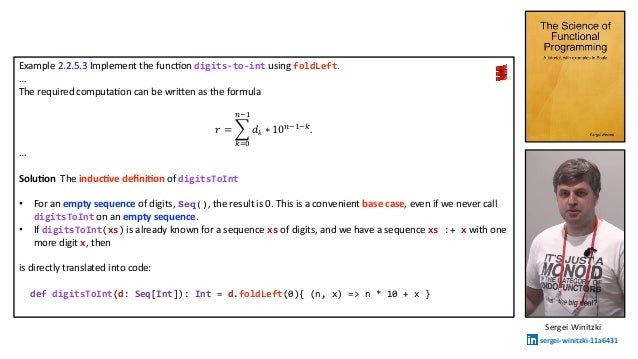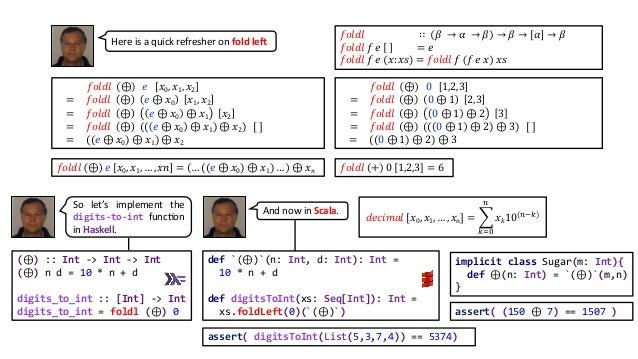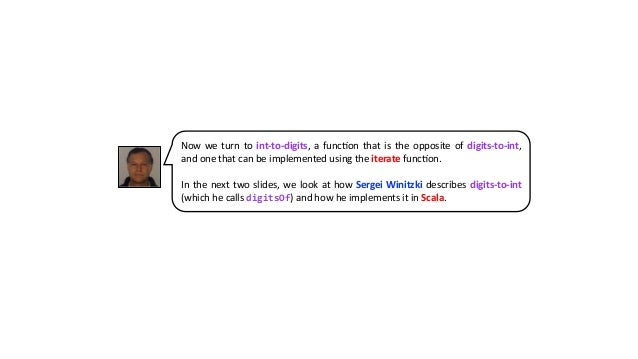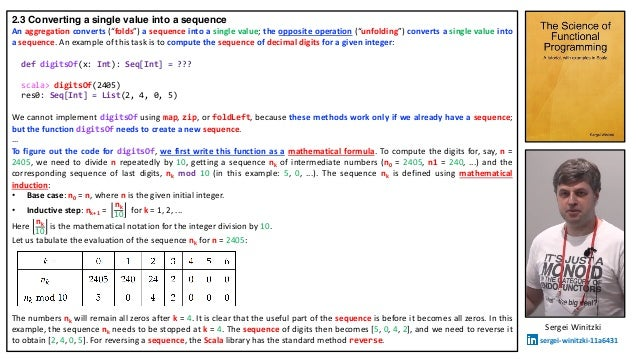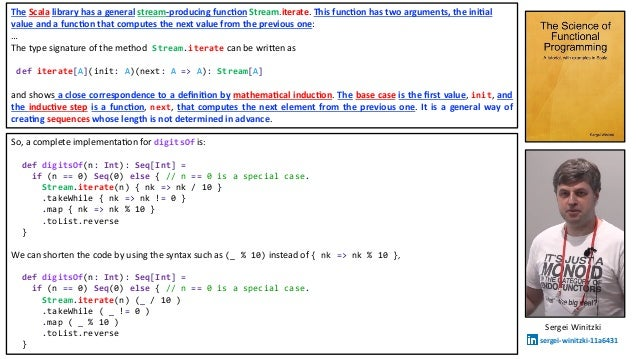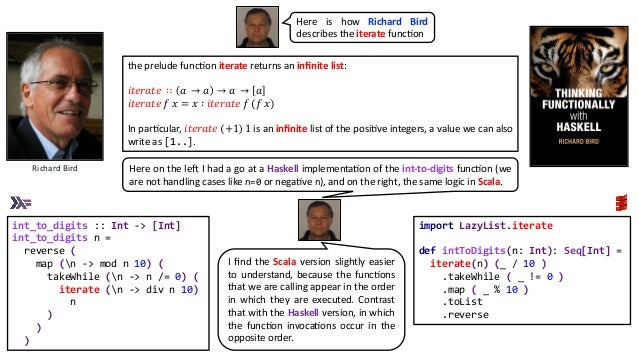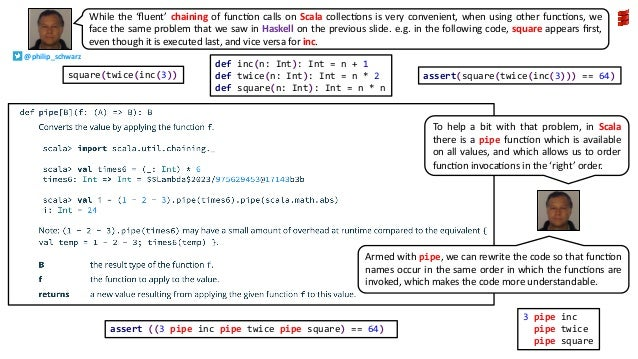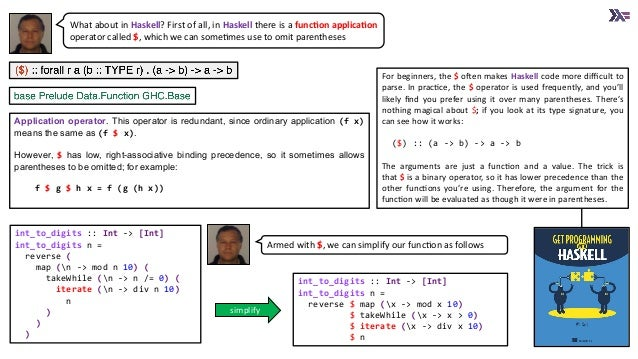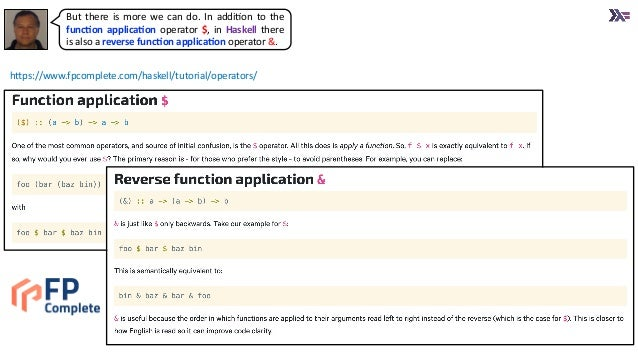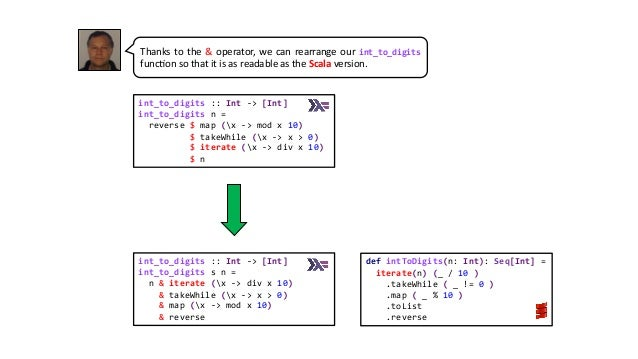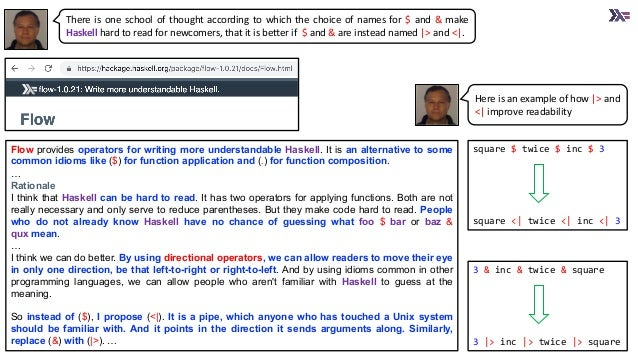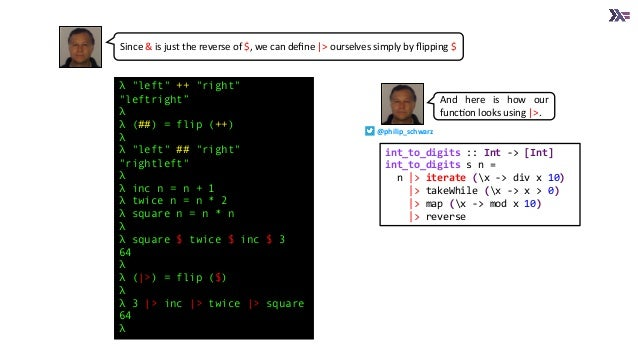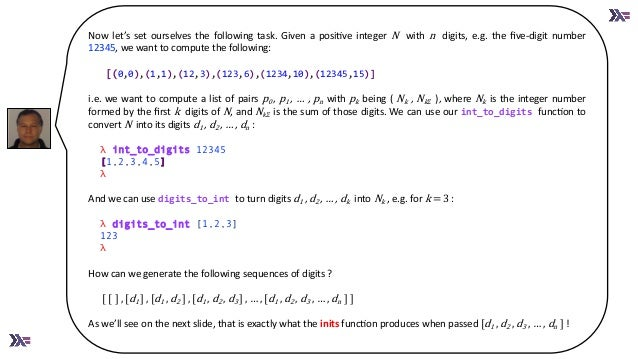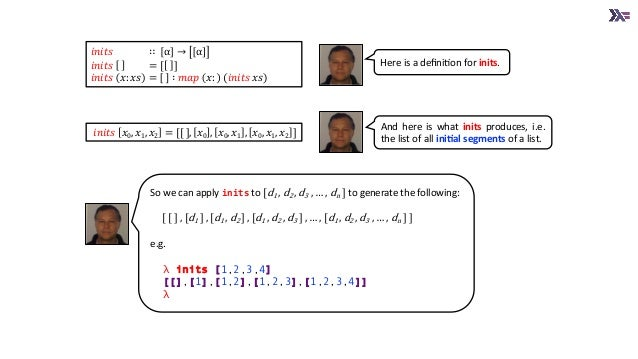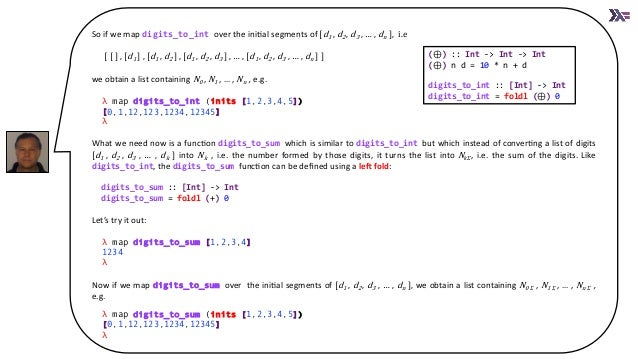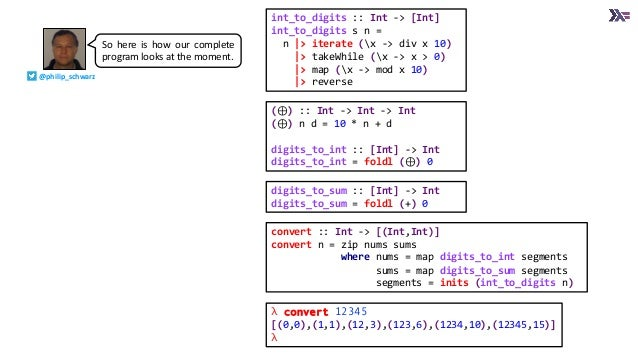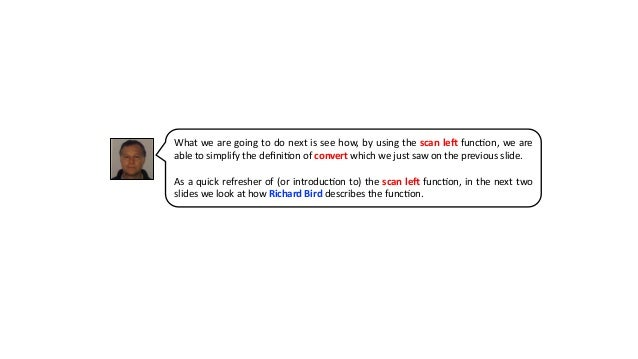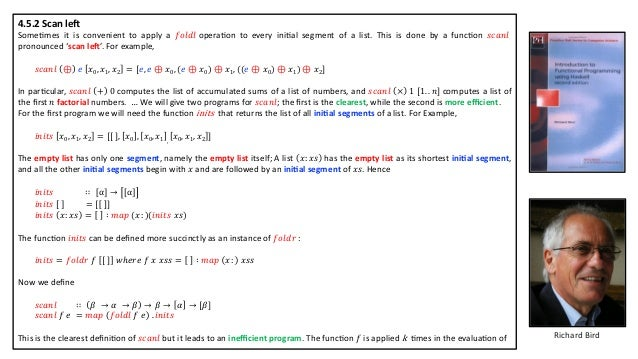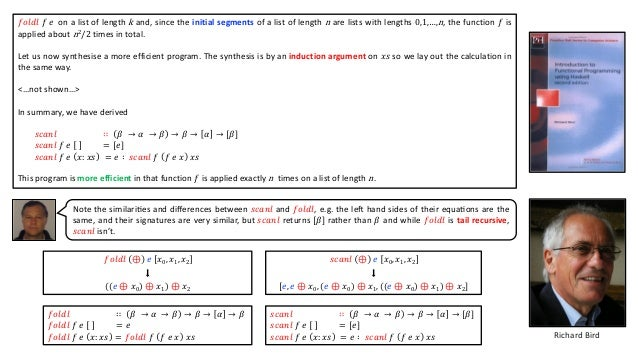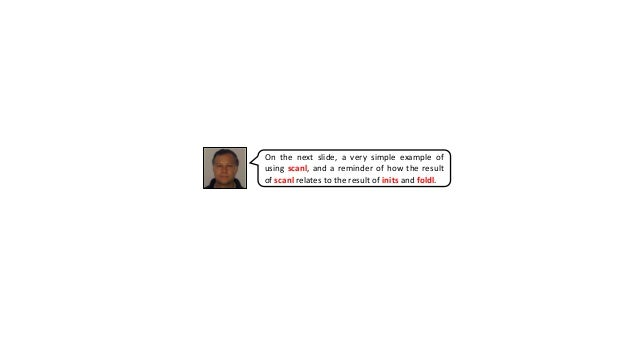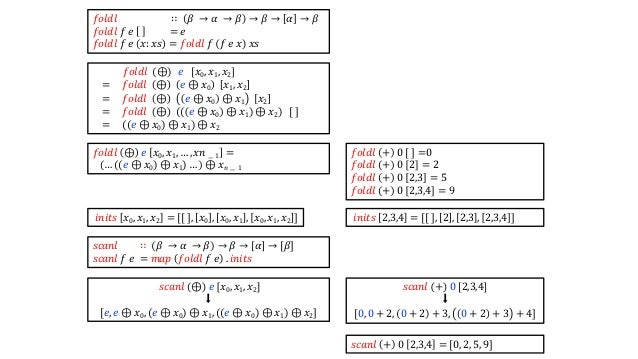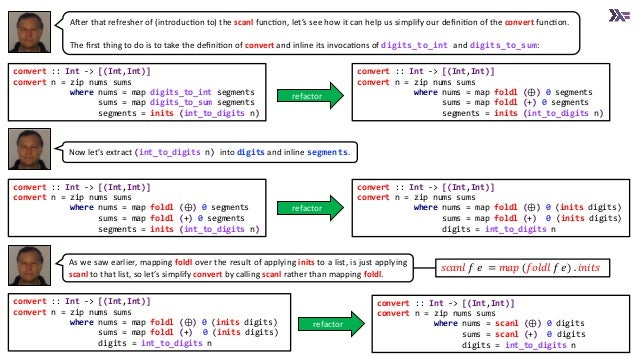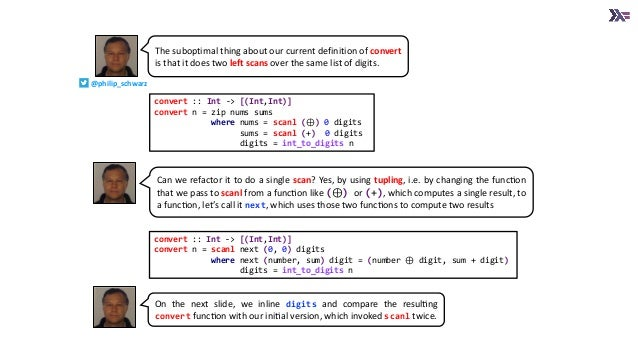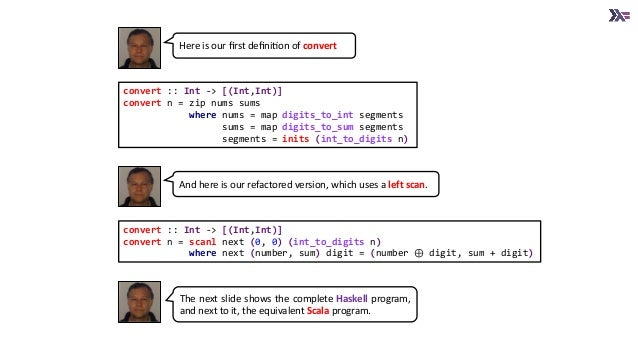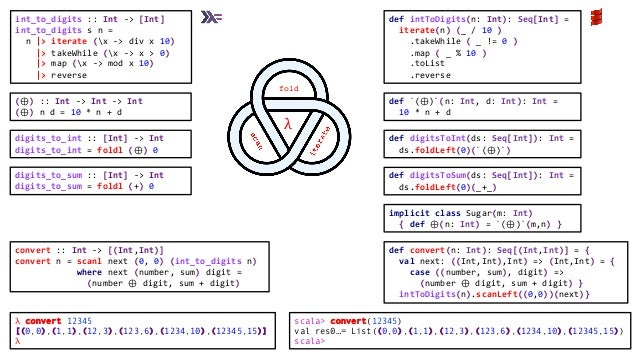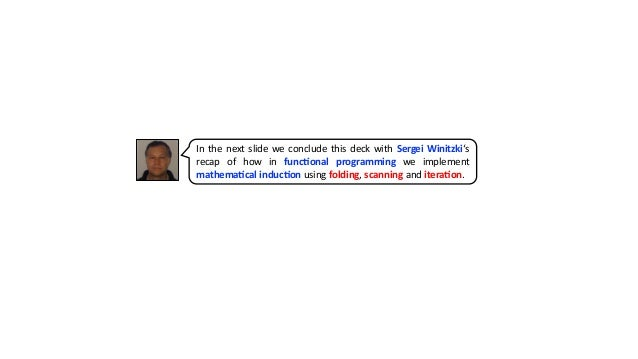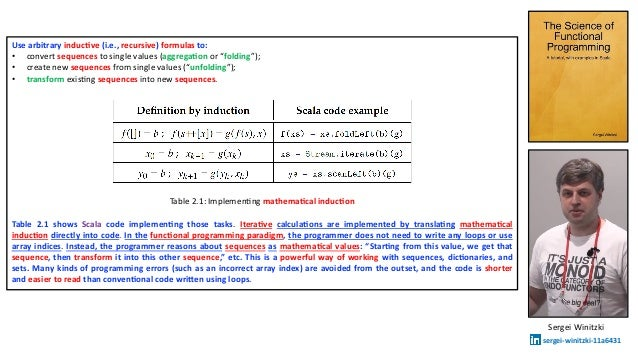Upcoming SlideShare
Loading in …5
×

# The Functional Programming Triad of Folding, Scanning and Iteration - a first example in Scala and Haskell - Polyglot FP for Fun and Profit

This slide deck can work both as an aide mémoire (memory jogger), or as a first (not completely trivial) example of using left folds, left scans and iteration, to implement mathematical induction.

Errata: on almost half of the slides there is some minor typo or imperfection or in some cases a minor error and in one case, an omission. See a later version for corrections and some improvements.

(for best quality images, either download or view here: https://philipschwarz.dev/fpilluminated/?page_id=455)

Scala code for latest version: https://github.com/philipschwarz/fp-fold-scan-iterate-triad-a-first-example-scala

• Full Name
Comment goes here.

Are you sure you want to Yes No
Your message goes here• Be the first to comment

### The Functional Programming Triad of Folding, Scanning and Iteration - a first example in Scala and Haskell - Polyglot FP for Fun and Profit

1. 1. fold λ The Func%onal Programming Triad of Folding, Scanning and Itera%on a ﬁrst example in Scala and Haskell Polyglot FP for Fun and Proﬁt @philip_schwarz slides by h"ps://www.slideshare.net/pjschwarz Richard Bird Sergei Winitzki sergei-winitzki-11a6431 http://www.cs.ox.ac.uk/people/richard.bird/
2. 2. This slide deck can work both as an aide mémoire (memory jogger), or as a ﬁrst (not completely trivial) example of using le/ folds, le/ scans and itera5on to implement mathema5cal induc5on. We ﬁrst look at the implementaAon, using a le/ fold, of a digits-to-int funcAon that converts a sequence of digits into a whole number. Then we look at the implementaAon, using the iterate funcAon, of an int-to- digits funcAon that converts an integer into a sequence of digits. In Scala this funcAon is very readable because the signatures of funcAons provided by collec5ons permit the piping of such funcAons with zero syntac5c overhead. In Haskell, there is some syntac5c sugar that can be used to achieve the same readability, so we look at how that works. We then set ourselves a simple task involving digits-to-int and int-to-digits, and write a funcAon whose logic can be simpliﬁed with the introducAon of a le/ scan. Let’s begin, on the next slide, by looking at how Richard Bird describes the digits- to-int funcAon, which he calls 𝑑𝑒𝑐𝑖𝑚𝑎𝑙. @philip_schwarz
3. 3. Suppose we want a funcAon decimal that takes a list of digits and returns the corresponding decimal number; thus 𝑑𝑒𝑐𝑖𝑚𝑎𝑙 [𝑥0, 𝑥1, … , 𝑥n] = ∑!"# \$ 𝑥 𝑘10(\$&!) It is assumed that the most signiﬁcant digit comes ﬁrst in the list. One way to compute decimal eﬃciently is by a process of mulAplying each digit by ten and adding in the following digit. For example 𝑑𝑒𝑐𝑖𝑚𝑎𝑙 𝑥0, 𝑥1, 𝑥2 = 10 × 10 × 10 × 0 + 𝑥0 + 𝑥1 + 𝑥2 This decomposiAon of a sum of powers is known as Horner’s rule. Suppose we deﬁne ⊕ by 𝑛 ⊕ 𝑥 = 10 × 𝑛 + 𝑥. Then we can rephrase the above equaAon as 𝑑𝑒𝑐𝑖𝑚𝑎𝑙 𝑥0, 𝑥1, 𝑥2 = (0 ⊕ 𝑥0) ⊕ 𝑥1 ⊕ 𝑥2 This example moAvates the introducAon of a second fold operator called 𝑓𝑜𝑙𝑑𝑙 (pronounced ‘fold leM’). Informally: 𝑓𝑜𝑙𝑑𝑙 ⊕ 𝑒 𝑥0, 𝑥1, … , 𝑥𝑛 − 1 = … ((𝑒 ⊕ 𝑥0) ⊕ 𝑥1) … ⊕ 𝑥 𝑛 − 1 The parentheses group from the leM, which is the reason for the name. The full deﬁniAon of 𝑓𝑜𝑙𝑑𝑙 is 𝑓𝑜𝑙𝑑𝑙 ∷ 𝛽 → 𝛼 → 𝛽 → 𝛽 → 𝛼 → 𝛽 𝑓𝑜𝑙𝑑𝑙 𝑓 𝑒 = 𝑒 𝑓𝑜𝑙𝑑𝑙 𝑓 𝑒 𝑥: 𝑥𝑠 = 𝑓𝑜𝑙𝑑𝑙 𝑓 𝑓 𝑒 𝑥 𝑥𝑠 Richard Bird
4. 4. On the next slide we look at how Sergei Winitzki describes the digits-to-int funcAon, and how he implements it in Scala.
5. 5. Example 2.2.5.3 Implement the funcAon digits-to-int using foldLeft. … The required computaAon can be wriTen as the formula 𝑟 = @ !"# \$&( 𝑑 𝑘 ∗ 10\$&(&!. … Solu5on The induc5ve deﬁni5on of digitsToInt • For an empty sequence of digits, Seq(), the result is 0. This is a convenient base case, even if we never call digitsToInt on an empty sequence. • If digitsToInt(xs) is already known for a sequence xs of digits, and we have a sequence xs :+ x with one more digit x, then is directly translated into code: def digitsToInt(d: Seq[Int]): Int = d.foldLeft(0){ (n, x) => n * 10 + x } Sergei Winitzki sergei-winitzki-11a6431
6. 6. (⊕) :: Int -> Int -> Int (⊕) n d = 10 * n + d digits_to_int :: [Int] -> Int digits_to_int = foldl (⊕) 0 def `(⊕)`(n: Int, d: Int): Int = 10 * n + d def digitsToInt(xs: Seq[Int]): Int = xs.foldLeft(0)(`(⊕)`) assert( digitsToInt(List(5,3,7,4)) == 5374) implicit class Sugar(m: Int){ def ⊕(n: Int) = `(⊕)`(m,n) } assert( (150 ⊕ 7) == 1507 ) 𝑓𝑜𝑙𝑑𝑙 + 0 1,2,3 = 6𝑓𝑜𝑙𝑑𝑙 ⊕ 𝑒 𝑥0, 𝑥1, … , 𝑥𝑛 = … ((𝑒 ⊕ 𝑥0) ⊕ 𝑥1) … ⊕ 𝑥 𝑛 𝑓𝑜𝑙𝑑𝑙 ∷ 𝛽 → 𝛼 → 𝛽 → 𝛽 → 𝛼 → 𝛽 𝑓𝑜𝑙𝑑𝑙 𝑓 𝑒 = 𝑒 𝑓𝑜𝑙𝑑𝑙 𝑓 𝑒 𝑥: 𝑥𝑠 = 𝑓𝑜𝑙𝑑𝑙 𝑓 𝑓 𝑒 𝑥 𝑥𝑠 𝑓𝑜𝑙𝑑𝑙 ⊕ 𝑒 𝑥0, 𝑥1, 𝑥2 = 𝑓𝑜𝑙𝑑𝑙 ⊕ 𝑒 ⊕ 𝑥0 𝑥1, 𝑥2 = 𝑓𝑜𝑙𝑑𝑙 ⊕ 𝑒 ⊕ 𝑥0 ⊕ 𝑥1 𝑥2 = 𝑓𝑜𝑙𝑑𝑙 ⊕ (( 𝑒 ⊕ 𝑥0 ⊕ 𝑥1) ⊕ 𝑥2) [ ] = ((𝑒 ⊕ 𝑥0) ⊕ 𝑥1) ⊕ 𝑥2 𝑓𝑜𝑙𝑑𝑙 ⊕ 0 1,2,3 = 𝑓𝑜𝑙𝑑𝑙 ⊕ 0 ⊕ 1 2,3 = 𝑓𝑜𝑙𝑑𝑙 ⊕ 0 ⊕ 1 ⊕ 2 3 = 𝑓𝑜𝑙𝑑𝑙 ⊕ (( 0 ⊕ 1 ⊕ 2) ⊕ 3) [ ] = ((0 ⊕ 1) ⊕ 2) ⊕ 3 Here is a quick refresher on fold le/ So let’s implement the digits-to-int funcAon in Haskell. And now in Scala. 𝑑𝑒𝑐𝑖𝑚𝑎𝑙 [𝑥0, 𝑥1, … , 𝑥n] = @ !"# \$ 𝑥 𝑘10(\$&!)
7. 7. Now we turn to int-to-digits, a funcAon that is the opposite of digits-to-int, and one that can be implemented using the iterate funcAon. In the next two slides, we look at how Sergei Winitzki describes digits-to-int (which he calls digitsOf) and how he implements it in Scala.
8. 8. 2.3 Converting a single value into a sequence An aggregation converts (“folds”) a sequence into a single value; the opposite operation (“unfolding”) converts a single value into a sequence. An example of this task is to compute the sequence of decimal digits for a given integer: def digitsOf(x: Int): Seq[Int] = ??? scala> digitsOf(2405) res0: Seq[Int] = List(2, 4, 0, 5) We cannot implement digitsOf using map, zip, or foldLeft, because these methods work only if we already have a sequence; but the function digitsOf needs to create a new sequence. … To figure out the code for digitsOf, we first write this function as a mathematical formula. To compute the digits for, say, n = 2405, we need to divide n repeatedly by 10, getting a sequence nk of intermediate numbers (n0 = 2405, n1 = 240, ...) and the corresponding sequence of last digits, nk mod 10 (in this example: 5, 0, ...). The sequence nk is defined using mathematical induction: • Base case: n0 = n, where n is the given initial integer. • Inductive step: nk+1 = nk 10 for k = 1, 2, ... Here nk 10 is the mathematical notation for the integer division by 10. Let us tabulate the evaluation of the sequence nk for n = 2405: The numbers nk will remain all zeros after k = 4. It is clear that the useful part of the sequence is before it becomes all zeros. In this example, the sequence nk needs to be stopped at k = 4. The sequence of digits then becomes [5, 0, 4, 2], and we need to reverse it to obtain [2, 4, 0, 5]. For reversing a sequence, the Scala library has the standard method reverse. Sergei Winitzki sergei-winitzki-11a6431
9. 9. So, a complete implementa.on for digitsOf is: def digitsOf(n: Int): Seq[Int] = if (n == 0) Seq(0) else { // n == 0 is a special case. Stream.iterate(n) { nk => nk / 10 } .takeWhile { nk => nk != 0 } .map { nk => nk % 10 } .toList.reverse } We can shorten the code by using the syntax such as (_ % 10) instead of { nk => nk % 10 }, def digitsOf(n: Int): Seq[Int] = if (n == 0) Seq(0) else { // n == 0 is a special case. Stream.iterate(n) (_ / 10 ) .takeWhile ( _ != 0 ) .map ( _ % 10 ) .toList.reverse } Sergei Winitzki sergei-winitzki-11a6431 The Scala library has a general stream-producing func8on Stream.iterate. This func8on has two arguments, the ini8al value and a func8on that computes the next value from the previous one: … The type signature of the method Stream.iterate can be wri?en as def iterate[A](init: A)(next: A => A): Stream[A] and shows a close correspondence to a deﬁni8on by mathema8cal induc8on. The base case is the ﬁrst value, init, and the induc8ve step is a func8on, next, that computes the next element from the previous one. It is a general way of crea8ng sequences whose length is not determined in advance.
10. 10. the prelude funcAon iterate returns an inﬁnite list: 𝑖𝑡𝑒𝑟𝑎𝑡𝑒 ∷ 𝑎 → 𝑎 → 𝑎 → 𝑎 𝑖𝑡𝑒𝑟𝑎𝑡𝑒 𝑓 𝑥 = 𝑥 ∶ 𝑖𝑡𝑒𝑟𝑎𝑡𝑒 𝑓 (𝑓 𝑥) In parAcular, 𝑖𝑡𝑒𝑟𝑎𝑡𝑒 +1 1 is an inﬁnite list of the posiAve integers, a value we can also write as [1..]. Richard Bird Here is how Richard Bird describes the iterate funcAon int_to_digits :: Int -> [Int] int_to_digits n = reverse ( map (n -> mod n 10) ( takeWhile (n -> n /= 0) ( iterate (n -> div n 10) n ) ) ) import LazyList.iterate def intToDigits(n: Int): Seq[Int] = iterate(n) (_ / 10 ) .takeWhile ( _ != 0 ) .map ( _ % 10 ) .toList .reverse Here on the leM I had a go at a Haskell implementaAon of the int-to-digits funcAon (we are not handling cases like n=0 or negaAve n), and on the right, the same logic in Scala. I ﬁnd the Scala version slightly easier to understand, because the funcAons that we are calling appear in the order in which they are executed. Contrast that with the Haskell version, in which the funcAon invocaAons occur in the opposite order.
11. 11. While the ‘ﬂuent’ chaining of funcAon calls on Scala collecAons is very convenient, when using other funcAons, we face the same problem that we saw in Haskell on the previous slide. e.g. in the following code, square appears ﬁrst, even though it is executed last, and vice versa for inc. assert(square(twice(inc(3))) == 64) assert ((3 pipe inc pipe twice pipe square) == 64) To help a bit with that problem, in Scala there is a pipe funcAon which is available on all values, and which allows us to order funcAon invocaAons in the ‘right’ order. Armed with pipe, we can rewrite the code so that funcAon names occur in the same order in which the funcAons are invoked, which makes the code more understandable. def inc(n: Int): Int = n + 1 def twice(n: Int): Int = n * 2 def square(n: Int): Int = n * n 3 pipe inc pipe twice pipe square square(twice(inc(3)) @philip_schwarz
12. 12. What about in Haskell? First of all, in Haskell there is a func8on applica8on operator called \$, which we can some.mes use to omit parentheses Application operator. This operator is redundant, since ordinary application (f x) means the same as (f \$ x). However, \$ has low, right-associative binding precedence, so it sometimes allows parentheses to be omitted; for example: f \$ g \$ h x = f (g (h x)) int_to_digits :: Int -> [Int] int_to_digits n = reverse ( map (n -> mod n 10) ( takeWhile (n -> n /= 0) ( iterate (n -> div n 10) n ) ) ) int_to_digits :: Int -> [Int] int_to_digits n = reverse \$ map (x -> mod x 10) \$ takeWhile (x -> x > 0) \$ iterate (x -> div x 10) \$ n Armed with \$, we can simplify our func.on as follows simplify For beginners, the \$ oVen makes Haskell code more diﬃcult to parse. In pracXce, the \$ operator is used frequently, and you’ll likely ﬁnd you prefer using it over many parentheses. There’s nothing magical about \$; if you look at its type signature, you can see how it works: (\$) :: (a -> b) -> a -> b The arguments are just a funcXon and a value. The trick is that \$ is a binary operator, so it has lower precedence than the other funcXons you’re using. Therefore, the argument for the funcXon will be evaluated as though it were in parentheses.
13. 13. But there is more we can do. In addiAon to the func5on applica5on operator \$, in Haskell there is also a reverse func5on applica5on operator &. hTps://www.fpcomplete.com/haskell/tutorial/operators/
14. 14. int_to_digits :: Int -> [Int] int_to_digits n = reverse \$ map (x -> mod x 10) \$ takeWhile (x -> x > 0) \$ iterate (x -> div x 10) \$ n int_to_digits :: Int -> [Int] int_to_digits s n = n & iterate (x -> div x 10) & takeWhile (x -> x > 0) & map (x -> mod x 10) & reverse def intToDigits(n: Int): Seq[Int] = iterate(n) (_ / 10 ) .takeWhile ( _ != 0 ) .map ( _ % 10 ) .toList .reverse Thanks to the & operator, we can rearrange our int_to_digits funcAon so that it is as readable as the Scala version.
15. 15. There is one school of thought according to which the choice of names for \$ and & make Haskell hard to read for newcomers, that it is beTer if \$ and & are instead named |> and <|. Flow provides operators for writing more understandable Haskell. It is an alternative to some common idioms like (\$) for function application and (.) for function composition. … Rationale I think that Haskell can be hard to read. It has two operators for applying functions. Both are not really necessary and only serve to reduce parentheses. But they make code hard to read. People who do not already know Haskell have no chance of guessing what foo \$ bar or baz & qux mean. … I think we can do better. By using directional operators, we can allow readers to move their eye in only one direction, be that left-to-right or right-to-left. And by using idioms common in other programming languages, we can allow people who aren't familiar with Haskell to guess at the meaning. So instead of (\$), I propose (<|). It is a pipe, which anyone who has touched a Unix system should be familiar with. And it points in the direction it sends arguments along. Similarly, replace (&) with (|>). … square \$ twice \$ inc \$ 3 square <| twice <| inc <| 3 3 & inc & twice & square 3 |> inc |> twice |> square Here is an example of how |> and <| improve readability
16. 16. Since & is just the reverse of \$, we can deﬁne |> ourselves simply by ﬂipping \$ λ "left" ++ "right" "leftright” λ λ (##) = flip (++) λ λ "left" ## "right" "rightleft" λ λ inc n = n + 1 λ twice n = n * 2 λ square n = n * n λ λ square \$ twice \$ inc \$ 3 64 λ λ (|>) = flip (\$) λ λ 3 |> inc |> twice |> square 64 λ int_to_digits :: Int -> [Int] int_to_digits s n = n |> iterate (x -> div x 10) |> takeWhile (x -> x > 0) |> map (x -> mod x 10) |> reverse And here is how our funcAon looks using |>. @philip_schwarz
17. 17. Now let’s set ourselves the following task. Given a posiAve integer N with n digits, e.g. the ﬁve-digit number 12345, we want to compute the following: [(0,0),(1,1),(12,3),(123,6),(1234,10),(12345,15)] i.e. we want to compute a list of pairs p0 , p1 , … , pn with pk being ( Nk , Nk 𝛴 ), where Nk is the integer number formed by the ﬁrst k digits of N, and Nk𝛴 is the sum of those digits. We can use our int_to_digits funcAon to convert N into its digits d1 , d2 , … , dn : λ int_to_digits 12345 [1,2,3,4,5] λ And we can use digits_to_int to turn digits d1 , d2 , … , dk into Nk , e.g. for k = 3 : λ digits_to_int [1,2,3] 123 λ How can we generate the following sequences of digits ? [ [ ] , [d1 ] , [d1 , d2 ] , [d1 , d2 , d3 ] , … , [d1 , d2 , d3 , … , dn ] ] As we’ll see on the next slide, that is exactly what the inits funcAon produces when passed [d1 , d2 , d3 , … , dn ] !
18. 18. 𝑖𝑛𝑖𝑡𝑠 𝑥0, 𝑥1, 𝑥2 = [[ ], 𝑥0 , 𝑥0, 𝑥1 , 𝑥0, 𝑥1, 𝑥2 ] 𝑖𝑛𝑖𝑡𝑠 ∷ α → α 𝑖𝑛𝑖𝑡𝑠 = [ ] 𝑖𝑛𝑖𝑡𝑠 𝑥: 𝑥𝑠 = ∶ 𝑚𝑎𝑝 𝑥: (𝑖𝑛𝑖𝑡𝑠 𝑥𝑠) Here is a deﬁniAon for inits. So we can apply inits to [d1 , d2 , d3 , … , dn ] to generate the following: [ [ ] , [d1 ] , [d1 , d2 ] , [d1 , d2 , d3 ] , … , [d1 , d2 , d3 , … , dn ] ] e.g. λ inits [1,2,3,4] [[],,[1,2],[1,2,3],[1,2,3,4]]] λ And here is what inits produces, i.e. the list of all ini5al segments of a list.
19. 19. So if we map digits_to_int over the iniXal segments of [d1 , d2 , d3 , … , dn ], i.e [ [ ] , [d1 ] , [d1 , d2 ] , [d1 , d2 , d3 ] , … , [d1 , d2 , d3 , … , dn ] ] we obtain a list containing N0 , N1 , … , Nn , e.g. λ map digits_to_int (inits [1,2,3,4,5])) [0,1,12,123,1234,12345]] λ What we need now is a funcXon digits_to_sum which is similar to digits_to_int but which instead of converXng a list of digits [d1 , d2 , d3 , … , dk ] into Nk , i.e. the number formed by those digits, it turns the list into Nk𝛴, i.e. the sum of the digits. Like digits_to_int, the digits_to_sum funcXon can be deﬁned using a leB fold: digits_to_sum :: [Int] -> Int digits_to_sum = foldl (+) 0 Let’s try it out: λ map digits_to_sum [1,2,3,4]) 1234] λ Now if we map digits_to_sum over the iniXal segments of [d1 , d2 , d3 , … , dn ], we obtain a list containing N0 𝛴 , N1 𝛴 , … , Nn 𝛴 , e.g. λ map digits_to_sum (inits [1,2,3,4,5])) [0,1,12,123,1234,12345]] λ (⊕) :: Int -> Int -> Int (⊕) n d = 10 * n + d digits_to_int :: [Int] -> Int digits_to_int = foldl (⊕) 0
20. 20. digits_to_sum :: [Int] -> Int digits_to_sum = foldl (+) 0 (⊕) :: Int -> Int -> Int (⊕) n d = 10 * n + d digits_to_int :: [Int] -> Int digits_to_int = foldl (⊕) 0 convert :: Int -> [(Int,Int)] convert n = zip nums sums where nums = map digits_to_int segments sums = map digits_to_sum segments segments = inits (int_to_digits n) int_to_digits :: Int -> [Int] int_to_digits s n = n |> iterate (x -> div x 10) |> takeWhile (x -> x > 0) |> map (x -> mod x 10) |> reverse λ convert 12345 [(0,0),(1,1),(12,3),(123,6),(1234,10),(12345,15)] λ So here is how our complete program looks at the moment. @philip_schwarz
21. 21. What we are going to do next is see how, by using the scan le/ funcAon, we are able to simplify the deﬁniAon of convert which we just saw on the previous slide. As a quick refresher of (or introducAon to) the scan le/ funcAon, in the next two slides we look at how Richard Bird describes the funcAon.
22. 22. 4.5.2 Scan le/ SomeXmes it is convenient to apply a 𝑓𝑜𝑙𝑑𝑙 operaXon to every iniXal segment of a list. This is done by a funcXon 𝑠𝑐𝑎𝑛𝑙 pronounced ‘scan leB’. For example, 𝑠𝑐𝑎𝑛𝑙 ⊕ 𝑒 𝑥0, 𝑥1, 𝑥2 = [𝑒, 𝑒 ⊕ 𝑥0, (𝑒 ⊕ 𝑥0) ⊕ 𝑥1, ((𝑒 ⊕ 𝑥0) ⊕ 𝑥1) ⊕ 𝑥2] In parXcular, 𝑠𝑐𝑎𝑛𝑙 + 0 computes the list of accumulated sums of a list of numbers, and 𝑠𝑐𝑎𝑛𝑙 × 1 [1. . 𝑛] computes a list of the ﬁrst 𝑛 factorial numbers. … We will give two programs for 𝑠𝑐𝑎𝑛𝑙; the ﬁrst is the clearest, while the second is more eﬃcient. For the ﬁrst program we will need the funcXon inits that returns the list of all iniDal segments of a list. For Example, 𝑖𝑛𝑖𝑡𝑠 𝑥0, 𝑥1, 𝑥2 = [[ ], 𝑥0 , 𝑥0, 𝑥1 , 𝑥0, 𝑥1, 𝑥2 ] The empty list has only one segment, namely the empty list itself; A list 𝑥: 𝑥𝑠 has the empty list as its shortest iniDal segment, and all the other iniDal segments begin with 𝑥 and are followed by an iniDal segment of 𝑥𝑠. Hence 𝑖𝑛𝑖𝑡𝑠 ∷ α → α 𝑖𝑛𝑖𝑡𝑠 = [ ] 𝑖𝑛𝑖𝑡𝑠 𝑥: 𝑥𝑠 = ∶ 𝑚𝑎𝑝 (𝑥: )(𝑖𝑛𝑖𝑡𝑠 𝑥𝑠) The funcXon 𝑖𝑛𝑖𝑡𝑠 can be deﬁned more succinctly as an instance of 𝑓𝑜𝑙𝑑𝑟 : 𝑖𝑛𝑖𝑡𝑠 = 𝑓𝑜𝑙𝑑𝑟 𝑓 [ ] 𝑤ℎ𝑒𝑟𝑒 𝑓 𝑥 𝑥𝑠𝑠 = ∶ 𝑚𝑎𝑝 𝑥: 𝑥𝑠𝑠 Now we deﬁne 𝑠𝑐𝑎𝑛𝑙 ∷ 𝛽 → 𝛼 → 𝛽 → 𝛽 → 𝛼 → [𝛽] 𝑠𝑐𝑎𝑛𝑙 𝑓 𝑒 = 𝑚𝑎𝑝 𝑓𝑜𝑙𝑑𝑙 𝑓 𝑒 . 𝑖𝑛𝑖𝑡𝑠 This is the clearest deﬁniXon of 𝑠𝑐𝑎𝑛𝑙 but it leads to an ineﬃcient program. The funcXon 𝑓 is applied k Xmes in the evaluaXon of Richard Bird
23. 23. 𝑓𝑜𝑙𝑑𝑙 𝑓 𝑒 on a list of length k and, since the initial segments of a list of length n are lists with lengths 0,1,…,n, the function 𝑓 is applied about n2/2 times in total. Let us now synthesise a more efficient program. The synthesis is by an induction argument on 𝑥𝑠 so we lay out the calculation in the same way. <…not shown…> In summary, we have derived 𝑠𝑐𝑎𝑛𝑙 ∷ 𝛽 → 𝛼 → 𝛽 → 𝛽 → 𝛼 → [𝛽] 𝑠𝑐𝑎𝑛𝑙 𝑓 𝑒 = [𝑒] 𝑠𝑐𝑎𝑛𝑙 𝑓 𝑒 𝑥: 𝑥𝑠 = 𝑒 ∶ 𝑠𝑐𝑎𝑛𝑙 𝑓 𝑓 𝑒 𝑥 𝑥𝑠 This program is more efficient in that function 𝑓 is applied exactly n times on a list of length n. Richard Bird 𝑠𝑐𝑎𝑛𝑙 ⊕ 𝑒 𝑥0, 𝑥1, 𝑥2 ⬇ [𝑒, 𝑒 ⊕ 𝑥0, (𝑒 ⊕ 𝑥0) ⊕ 𝑥1, ((𝑒 ⊕ 𝑥0) ⊕ 𝑥1) ⊕ 𝑥2] 𝑓𝑜𝑙𝑑𝑙 ∷ 𝛽 → 𝛼 → 𝛽 → 𝛽 → 𝛼 → 𝛽 𝑓𝑜𝑙𝑑𝑙 𝑓 𝑒 = 𝑒 𝑓𝑜𝑙𝑑𝑙 𝑓 𝑒 𝑥: 𝑥𝑠 = 𝑓𝑜𝑙𝑑𝑙 𝑓 𝑓 𝑒 𝑥 𝑥𝑠 Note the similariXes and diﬀerences between 𝑠𝑐𝑎𝑛𝑙 and 𝑓𝑜𝑙𝑑𝑙, e.g. the leV hand sides of their equaXons are the same, and their signatures are very similar, but 𝑠𝑐𝑎𝑛𝑙 returns [𝛽] rather than 𝛽 and while 𝑓𝑜𝑙𝑑𝑙 is tail recursive, 𝑠𝑐𝑎𝑛𝑙 isn’t. 𝑠𝑐𝑎𝑛𝑙 ∷ 𝛽 → 𝛼 → 𝛽 → 𝛽 → 𝛼 → [𝛽] 𝑠𝑐𝑎𝑛𝑙 𝑓 𝑒 = [𝑒] 𝑠𝑐𝑎𝑛𝑙 𝑓 𝑒 𝑥: 𝑥𝑠 = 𝑒 ∶ 𝑠𝑐𝑎𝑛𝑙 𝑓 𝑓 𝑒 𝑥 𝑥𝑠 𝑓𝑜𝑙𝑑𝑙 ⊕ 𝑒 𝑥0, 𝑥1, 𝑥2 ⬇ ((𝑒 ⊕ 𝑥0) ⊕ 𝑥1) ⊕ 𝑥2
24. 24. On the next slide, a very simple example of using scanl, and a reminder of how the result of scanl relates to the result of inits and foldl.
25. 25. 𝑖𝑛𝑖𝑡𝑠 2,3,4 = [[ ], 2 , 2,3 , 2,3,4 ] 𝑠𝑐𝑎𝑛𝑙 + 0 2,3,4 = [0, 2, 5, 9] 𝑓𝑜𝑙𝑑𝑙 + 0 [ ] =0 𝑓𝑜𝑙𝑑𝑙 + 0 2 = 2 𝑓𝑜𝑙𝑑𝑙 + 0 2,3 = 5 𝑓𝑜𝑙𝑑𝑙 + 0 2,3,4 = 9 𝑠𝑐𝑎𝑛𝑙 ⊕ 𝑒 𝑥0, 𝑥1, 𝑥2 ⬇ [𝑒, 𝑒 ⊕ 𝑥0, (𝑒 ⊕ 𝑥0) ⊕ 𝑥1, ((𝑒 ⊕ 𝑥0) ⊕ 𝑥1) ⊕ 𝑥2] 𝑖𝑛𝑖𝑡𝑠 𝑥0, 𝑥1, 𝑥2 = [[ ], 𝑥0 , 𝑥0, 𝑥1 , 𝑥0, 𝑥1, 𝑥2 ] 𝑠𝑐𝑎𝑛𝑙 ∷ 𝛽 → 𝛼 → 𝛽 → 𝛽 → 𝛼 → [𝛽] 𝑠𝑐𝑎𝑛𝑙 𝑓 𝑒 = 𝑚𝑎𝑝 𝑓𝑜𝑙𝑑𝑙 𝑓 𝑒 . 𝑖𝑛𝑖𝑡𝑠 𝑓𝑜𝑙𝑑𝑙 ⊕ 𝑒 𝑥0, 𝑥1, … , 𝑥𝑛 − 1 = … ((𝑒 ⊕ 𝑥0) ⊕ 𝑥1) … ⊕ 𝑥 𝑛 − 1 𝑓𝑜𝑙𝑑𝑙 ∷ 𝛽 → 𝛼 → 𝛽 → 𝛽 → 𝛼 → 𝛽 𝑓𝑜𝑙𝑑𝑙 𝑓 𝑒 = 𝑒 𝑓𝑜𝑙𝑑𝑙 𝑓 𝑒 𝑥: 𝑥𝑠 = 𝑓𝑜𝑙𝑑𝑙 𝑓 𝑓 𝑒 𝑥 𝑥𝑠 𝑓𝑜𝑙𝑑𝑙 ⊕ 𝑒 𝑥0, 𝑥1, 𝑥2 = 𝑓𝑜𝑙𝑑𝑙 ⊕ 𝑒 ⊕ 𝑥0 𝑥1, 𝑥2 = 𝑓𝑜𝑙𝑑𝑙 ⊕ 𝑒 ⊕ 𝑥0 ⊕ 𝑥1 𝑥2 = 𝑓𝑜𝑙𝑑𝑙 ⊕ (( 𝑒 ⊕ 𝑥0 ⊕ 𝑥1) ⊕ 𝑥2) [ ] = ((𝑒 ⊕ 𝑥0) ⊕ 𝑥1) ⊕ 𝑥2 𝑠𝑐𝑎𝑛𝑙 + 0 2,3,4 ⬇ [0, 0 + 2, 0 + 2 + 3, 0 + 2 + 3 + 4]
26. 26. 𝑠𝑐𝑎𝑛𝑙 𝑓 𝑒 = 𝑚𝑎𝑝 𝑓𝑜𝑙𝑑𝑙 𝑓 𝑒 . 𝑖𝑛𝑖𝑡𝑠 convert :: Int -> [(Int,Int)] convert n = zip nums sums where nums = map digits_to_int segments sums = map digits_to_sum segments segments = inits (int_to_digits n) convert :: Int -> [(Int,Int)] convert n = zip nums sums where nums = map foldl (⊕) 0 segments sums = map foldl (+) 0 segments segments = inits (int_to_digits n) AVer that refresher of (introducXon to) the scanl funcXon, let’s see how it can help us simplify our deﬁniXon of the convert funcXon. The ﬁrst thing to do is to take the deﬁniXon of convert and inline its invocaXons of digits_to_int and digits_to_sum: refactor Now let’s extract (int_to_digits n) into digits and inline segments. refactor convert :: Int -> [(Int,Int)] convert n = zip nums sums where nums = map foldl (⊕) 0 (inits digits) sums = map foldl (+) 0 (inits digits) digits = int_to_digits n convert :: Int -> [(Int,Int)] convert n = zip nums sums where nums = map foldl (⊕) 0 segments sums = map foldl (+) 0 segments segments = inits (int_to_digits n) convert :: Int -> [(Int,Int)] convert n = zip nums sums where nums = scanl (⊕) 0 digits sums = scanl (+) 0 digits digits = int_to_digits n convert :: Int -> [(Int,Int)] convert n = zip nums sums where nums = map foldl (⊕) 0 (inits digits) sums = map foldl (+) 0 (inits digits) digits = int_to_digits n refactor As we saw earlier, mapping foldl over the result of applying inits to a list, is just applying scanl to that list, so let’s simplify convert by calling scanl rather than mapping foldl.
27. 27. convert :: Int -> [(Int,Int)] convert n = zip nums sums where nums = scanl (⊕) 0 digits sums = scanl (+) 0 digits digits = int_to_digits n The suboptimal thing about our current definition of convert is that it does two left scans over the same list of digits. Can we refactor it to do a single scan? Yes, by using tupling, i.e. by changing the func.on that we pass to scanl from a func.on like (⊕) or (+), which computes a single result, to a func.on, let’s call it next, which uses those two func.ons to compute two results convert :: Int -> [(Int,Int)] convert n = scanl next (0, 0) digits where next (number, sum) digit = (number ⊕ digit, sum + digit) digits = int_to_digits n On the next slide, we inline digits and compare the resul.ng convert func.on with our ini.al version, which invoked scanl twice. @philip_schwarz
28. 28. convert :: Int -> [(Int,Int)] convert n = scanl next (0, 0) (int_to_digits n) where next (number, sum) digit = (number ⊕ digit, sum + digit) convert :: Int -> [(Int,Int)] convert n = zip nums sums where nums = map digits_to_int segments sums = map digits_to_sum segments segments = inits (int_to_digits n) Here is our ﬁrst deﬁniAon of convert And here is our refactored version, which uses a left scan. The next slide shows the complete Haskell program, and next to it, the equivalent Scala program.
29. 29. digits_to_sum :: [Int] -> Int digits_to_sum = foldl (+) 0 (⊕) :: Int -> Int -> Int (⊕) n d = 10 * n + d int_to_digits :: Int -> [Int] int_to_digits s n = n |> iterate (x -> div x 10) |> takeWhile (x -> x > 0) |> map (x -> mod x 10) |> reverse λ convert 12345 [(0,0),(1,1),(12,3),(123,6),(1234,10),(12345,15)] λ convert :: Int -> [(Int,Int)] convert n = scanl next (0, 0) (int_to_digits n) where next (number, sum) digit = (number ⊕ digit, sum + digit) def digitsToInt(ds: Seq[Int]): Int = ds.foldLeft(0)(`(⊕)`) implicit class Sugar(m: Int) { def ⊕(n: Int) = `(⊕)`(m,n) } def digitsToSum(ds: Seq[Int]): Int = ds.foldLeft(0)(_+_) def intToDigits(n: Int): Seq[Int] = iterate(n) (_ / 10 ) .takeWhile ( _ != 0 ) .map ( _ % 10 ) .toList .reverse def convert(n: Int): Seq[(Int,Int)] = { val next: ((Int,Int),Int) => (Int,Int) = { case ((number, sum), digit) => (number ⊕ digit, sum + digit) } intToDigits(n).scanLeft((0,0))(next)} digits_to_int :: [Int] -> Int digits_to_int = foldl (⊕) 0 def `(⊕)`(n: Int, d: Int): Int = 10 * n + d scala> convert(12345) val res0…= List((0,0),(1,1),(12,3),(123,6),(1234,10),(12345,15)) scala> fold λ
30. 30. In the next slide we conclude this deck with Sergei Winitzki‘s recap of how in func5onal programming we implement mathema5cal induc5on using folding, scanning and itera5on.
31. 31. Use arbitrary inducDve (i.e., recursive) formulas to: • convert sequences to single values (aggregaDon or “folding”); • create new sequences from single values (“unfolding”); • transform exisXng sequences into new sequences. Table 2.1: ImplemenXng mathemaDcal inducDon Table 2.1 shows Scala code implemenDng those tasks. IteraDve calculaDons are implemented by translaDng mathemaDcal inducDon directly into code. In the funcDonal programming paradigm, the programmer does not need to write any loops or use array indices. Instead, the programmer reasons about sequences as mathemaDcal values: “StarDng from this value, we get that sequence, then transform it into this other sequence,” etc. This is a powerful way of working with sequences, dicDonaries, and sets. Many kinds of programming errors (such as an incorrect array index) are avoided from the outset, and the code is shorter and easier to read than convenDonal code wriPen using loops. Sergei Winitzki sergei-winitzki-11a6431